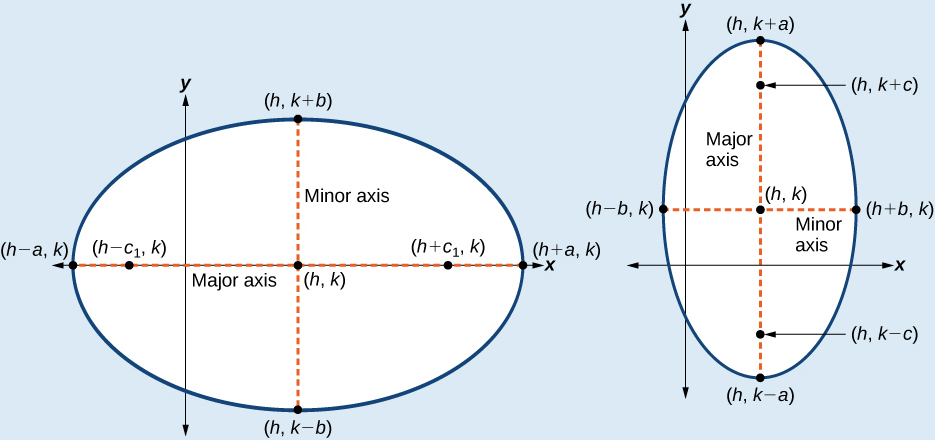# 12.1 The ellipse  (Page 4/16)

 Page 4 / 16

## Standard forms of the equation of an ellipse with center ( h , k )

The standard form of the equation of an ellipse with center and major axis    parallel to the x -axis is

$\frac{{\left(x-h\right)}^{2}}{{a}^{2}}+\frac{{\left(y-k\right)}^{2}}{{b}^{2}}=1$

where

• $a>b$
• the length of the major axis is $\text{\hspace{0.17em}}2a$
• the coordinates of the vertices are $\text{\hspace{0.17em}}\left(h±a,k\right)$
• the length of the minor axis is $\text{\hspace{0.17em}}2b$
• the coordinates of the co-vertices are $\text{\hspace{0.17em}}\left(h,k±b\right)$
• the coordinates of the foci are $\text{\hspace{0.17em}}\left(h±c,k\right),$ where $\text{\hspace{0.17em}}{c}^{2}={a}^{2}-{b}^{2}.\text{\hspace{0.17em}}$ See [link] a

The standard form of the equation of an ellipse with center $\text{\hspace{0.17em}}\left(h,k\right)\text{\hspace{0.17em}}$ and major axis parallel to the y -axis is

$\frac{{\left(x-h\right)}^{2}}{{b}^{2}}+\frac{{\left(y-k\right)}^{2}}{{a}^{2}}=1$

where

• $a>b$
• the length of the major axis is $\text{\hspace{0.17em}}2a$
• the coordinates of the vertices are $\text{\hspace{0.17em}}\left(h,k±a\right)$
• the length of the minor axis is $\text{\hspace{0.17em}}2b$
• the coordinates of the co-vertices are $\text{\hspace{0.17em}}\left(h±b,k\right)$
• the coordinates of the foci are $\text{\hspace{0.17em}}\left(h,k±c\right),\text{\hspace{0.17em}}$ where $\text{\hspace{0.17em}}{c}^{2}={a}^{2}-{b}^{2}.\text{\hspace{0.17em}}$ See [link] b

Just as with ellipses centered at the origin, ellipses that are centered at a point $\text{\hspace{0.17em}}\left(h,k\right)\text{\hspace{0.17em}}$ have vertices, co-vertices, and foci that are related by the equation $\text{\hspace{0.17em}}{c}^{2}={a}^{2}-{b}^{2}.\text{\hspace{0.17em}}$ We can use this relationship along with the midpoint and distance formulas to find the equation of the ellipse in standard form when the vertices and foci are given.(a) Horizontal ellipse with center   ( h , k )   (b) Vertical ellipse with center   ( h , k )

Given the vertices and foci of an ellipse not centered at the origin, write its equation in standard form.

1. Determine whether the major axis is parallel to the x - or y -axis.
1. If the y -coordinates of the given vertices and foci are the same, then the major axis is parallel to the x -axis. Use the standard form $\text{\hspace{0.17em}}\frac{{\left(x-h\right)}^{2}}{{a}^{2}}+\frac{{\left(y-k\right)}^{2}}{{b}^{2}}=1.$
2. If the x -coordinates of the given vertices and foci are the same, then the major axis is parallel to the y -axis. Use the standard form $\text{\hspace{0.17em}}\frac{{\left(x-h\right)}^{2}}{{b}^{2}}+\frac{{\left(y-k\right)}^{2}}{{a}^{2}}=1.$
2. Identify the center of the ellipse $\text{\hspace{0.17em}}\left(h,k\right)\text{\hspace{0.17em}}$ using the midpoint formula and the given coordinates for the vertices.
3. Find $\text{\hspace{0.17em}}{a}^{2}\text{\hspace{0.17em}}$ by solving for the length of the major axis, $\text{\hspace{0.17em}}2a,$ which is the distance between the given vertices.
4. Find $\text{\hspace{0.17em}}{c}^{2}\text{\hspace{0.17em}}$ using $\text{\hspace{0.17em}}h\text{\hspace{0.17em}}$ and $\text{\hspace{0.17em}}k,$ found in Step 2, along with the given coordinates for the foci.
5. Solve for $\text{\hspace{0.17em}}{b}^{2}\text{\hspace{0.17em}}$ using the equation $\text{\hspace{0.17em}}{c}^{2}={a}^{2}-{b}^{2}.$
6. Substitute the values for $\text{\hspace{0.17em}}h,k,{a}^{2},$ and $\text{\hspace{0.17em}}{b}^{2}\text{\hspace{0.17em}}$ into the standard form of the equation determined in Step 1.

## Writing the equation of an ellipse centered at a point other than the origin

What is the standard form equation of the ellipse that has vertices $\text{\hspace{0.17em}}\left(-2,-8\right)\text{\hspace{0.17em}}$ and $\text{\hspace{0.17em}}\left(-2,\text{2}\right)$

and foci $\text{\hspace{0.17em}}\left(-2,-7\right)\text{\hspace{0.17em}}$ and $\text{\hspace{0.17em}}\left(-2,\text{1}\right)?$

The x -coordinates of the vertices and foci are the same, so the major axis is parallel to the y -axis. Thus, the equation of the ellipse will have the form

$\frac{{\left(x-h\right)}^{2}}{{b}^{2}}+\frac{{\left(y-k\right)}^{2}}{{a}^{2}}=1$

First, we identify the center, $\text{\hspace{0.17em}}\left(h,k\right).\text{\hspace{0.17em}}$ The center is halfway between the vertices, $\text{\hspace{0.17em}}\left(-2,-8\right)\text{\hspace{0.17em}}$ and $\text{\hspace{0.17em}}\left(-2,\text{2}\right).\text{\hspace{0.17em}}$ Applying the midpoint formula, we have:

Next, we find $\text{\hspace{0.17em}}{a}^{2}.\text{\hspace{0.17em}}$ The length of the major axis, $\text{\hspace{0.17em}}2a,$ is bounded by the vertices. We solve for $\text{\hspace{0.17em}}a\text{\hspace{0.17em}}$ by finding the distance between the y -coordinates of the vertices.

$\begin{array}{c}\text{\hspace{0.17em}}\text{\hspace{0.17em}}\text{\hspace{0.17em}}\text{\hspace{0.17em}}\text{\hspace{0.17em}}\text{\hspace{0.17em}}\text{\hspace{0.17em}}\text{\hspace{0.17em}}\text{\hspace{0.17em}}\text{\hspace{0.17em}}\text{\hspace{0.17em}}\text{\hspace{0.17em}}\text{\hspace{0.17em}}\text{\hspace{0.17em}}\text{\hspace{0.17em}}\text{\hspace{0.17em}}2a=2-\left(-8\right)\\ 2a=10\\ a=5\end{array}$

So $\text{\hspace{0.17em}}{a}^{2}=25.$

Now we find $\text{\hspace{0.17em}}{c}^{2}.\text{\hspace{0.17em}}$ The foci are given by $\text{\hspace{0.17em}}\left(h,k±c\right).\text{\hspace{0.17em}}$ So, $\text{\hspace{0.17em}}\left(h,k-c\right)=\left(-2,-7\right)\text{\hspace{0.17em}}$ and $\text{\hspace{0.17em}}\left(h,k+c\right)=\left(-2,\text{1}\right).\text{\hspace{0.17em}}$ We substitute $\text{\hspace{0.17em}}k=-3\text{\hspace{0.17em}}$ using either of these points to solve for $\text{\hspace{0.17em}}c.$

$\begin{array}{c}\text{\hspace{0.17em}}\text{\hspace{0.17em}}\text{\hspace{0.17em}}\text{\hspace{0.17em}}k+c=1\\ -3+c=1\\ \text{\hspace{0.17em}}\text{\hspace{0.17em}}\text{\hspace{0.17em}}\text{\hspace{0.17em}}\text{\hspace{0.17em}}\text{\hspace{0.17em}}\text{\hspace{0.17em}}\text{\hspace{0.17em}}\text{\hspace{0.17em}}\text{\hspace{0.17em}}\text{\hspace{0.17em}}\text{\hspace{0.17em}}\text{\hspace{0.17em}}\text{\hspace{0.17em}}c=4\end{array}$

So $\text{\hspace{0.17em}}{c}^{2}=16.$

Next, we solve for $\text{\hspace{0.17em}}{b}^{2}\text{\hspace{0.17em}}$ using the equation $\text{\hspace{0.17em}}{c}^{2}={a}^{2}-{b}^{2}.$

$\begin{array}{c}\text{\hspace{0.17em}}\text{\hspace{0.17em}}\text{\hspace{0.17em}}\text{\hspace{0.17em}}\text{\hspace{0.17em}}\text{\hspace{0.17em}}\text{\hspace{0.17em}}\text{\hspace{0.17em}}\text{\hspace{0.17em}}\text{\hspace{0.17em}}\text{\hspace{0.17em}}\text{\hspace{0.17em}}\text{\hspace{0.17em}}\text{\hspace{0.17em}}{c}^{2}={a}^{2}-{b}^{2}\\ \text{\hspace{0.17em}}\text{\hspace{0.17em}}\text{\hspace{0.17em}}\text{\hspace{0.17em}}\text{\hspace{0.17em}}\text{\hspace{0.17em}}\text{\hspace{0.17em}}\text{\hspace{0.17em}}\text{\hspace{0.17em}}\text{\hspace{0.17em}}\text{\hspace{0.17em}}\text{\hspace{0.17em}}\text{\hspace{0.17em}}\text{\hspace{0.17em}}16=25-{b}^{2}\\ {b}^{2}=9\end{array}$

Finally, we substitute the values found for $\text{\hspace{0.17em}}h,k,{a}^{2},$ and $\text{\hspace{0.17em}}{b}^{2}\text{\hspace{0.17em}}$ into the standard form equation for an ellipse:

$\text{\hspace{0.17em}}\frac{{\left(x+2\right)}^{2}}{9}+\frac{{\left(y+3\right)}^{2}}{25}=1$

use the properties of logarithms to rewrite as a single logarithmic expression 2+log base 5 t divided by log base 5 17
(cos²x +tan²x)÷(sec²x+cos²x)
Solve the problem 3x^2_2x+4=0
can anyone recommend an app or. website to help me refresh my knowledge and abilities? I stopped learning more about calc and trig in the 90's
if 6x=-2 find value of 6/x
l don't know
Aman
I don't know
Aman
l don't know
Aman
I don't know
Aman
x=.333333333334, so 6/x= 18±
scott
x=.33333333334± 6/x=18±
scott
that is strange, I don't remember inserting the À. the result I got was x= 3333333334± 6/x=18±
scott
I need glasses
scott
X=(-1÷3) X equals minus one third
Melvin
how can I get the answer if don't know it
x=-3
Dani
-18
Nwaneri
if sin15°=√p, express the following in terms of p
I am learning and does someone have the time to tell me what or where this particular equation would be used?
scott
prove sin²x+cos²x=3+cos4x
the difference between two signed numbers is -8.if the minued is 5,what is the subtrahend
the difference between two signed numbers is -8.if the minuend is 5.what is the subtrahend
jeramie
what are odd numbers
numbers that leave a remainder when divided by 2
Thorben
1,3,5,7,... 99,...867
Thorben
7%2=1, 679%2=1, 866245%2=1
Thorben
the third and the seventh terms of a G.P are 81 and 16, find the first and fifth terms.
if a=3, b =4 and c=5 find the six trigonometric value sin
Ans
pls how do I factorize x⁴+x³-7x²-x+6=0
in a function the input value is called
how do I test for values on the number line
if a=4 b=4 then a+b=
a+b+2ab
Kin
commulative principle
a+b= 4+4=8
Mimi
If a=4 and b=4 then we add the value of a and b i.e a+b=4+4=8.
Tariq

#### Get Jobilize Job Search Mobile App in your pocket Now!ByByByBy Brooke DelaneyBy Joanna SmithbackBy OpenStaxBy Jonathan LongBy OpenStaxBy OpenStaxBy JavaChamp TeamBy OpenStaxBy Sarah Warren